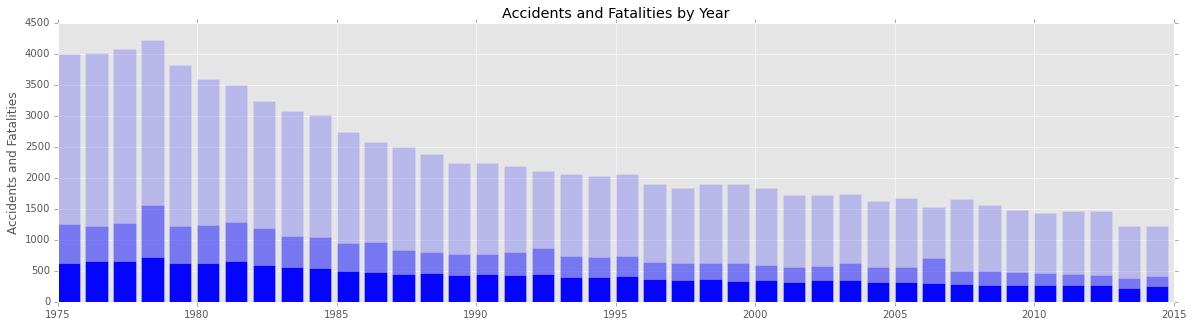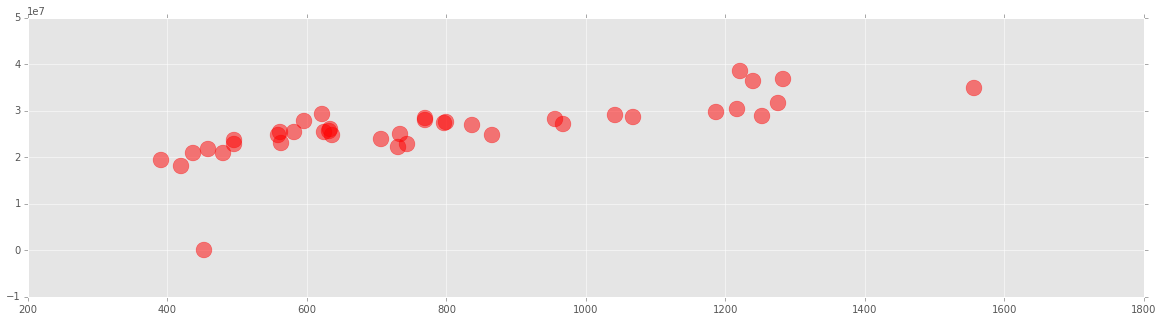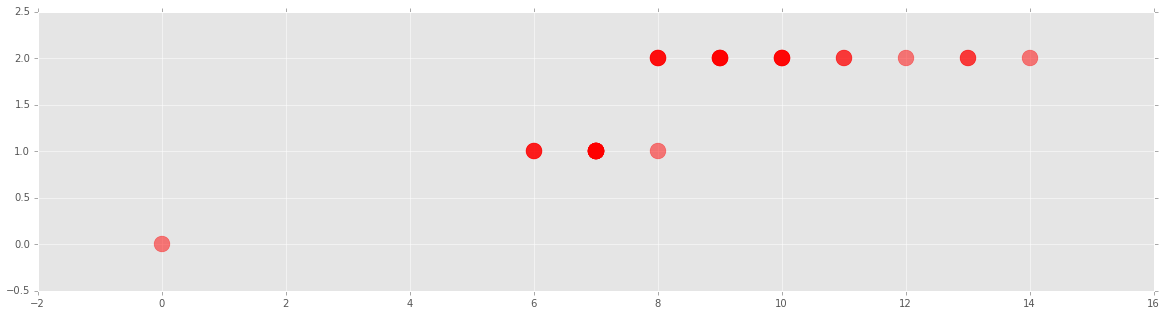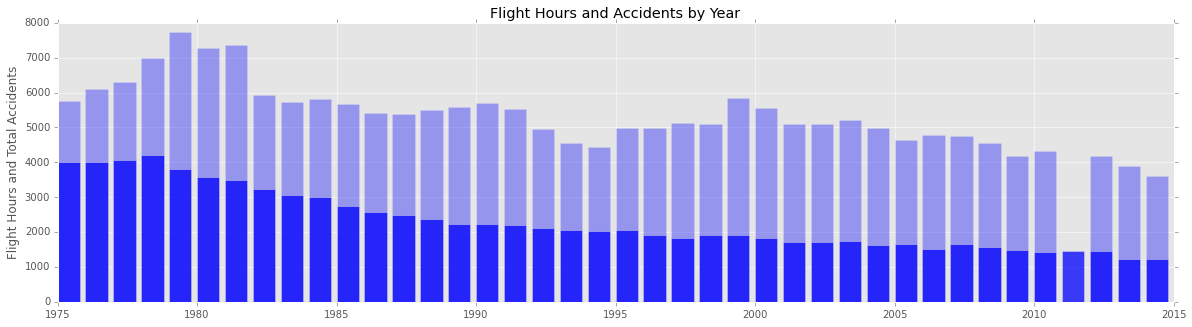In :
import pandas as pd
import numpy as np
%matplotlib inline
import matplotlib as mpl
mpl.style.use("ggplot")
import matplotlib.pyplot as plt
import ggplot
from ggplot import *


Here we have imported flight accident data from data.gov

In :
#Read the csv file into a local variable to create a data frame

In :
flight_df.head(5)

Out:
Year Total Accidents Fatal Accidents Total Fatalities Fatalities On Board Flight Hours AAP 100,000 Flight Hours FAP 100,000 Flight Hours
0 1975 3995 633 1252 1231 28799000 14 2
1 1976 4018 658 1216 1203 30476000 13 2
2 1977 4079 661 1276 1265 31578000 13 2
3 1978 4216 719 1556 1398 34887000 12 2
4 1979 3818 631 1221 1203 38641000 10 2
In :
plt.figure(figsize=(20,5))
plt.bar(flight_df["Year"], flight_df["Total Accidents"], alpha=0.2, color = "blue")
plt.bar(flight_df["Year"], flight_df["Fatal Accidents"], alpha=0.95, color = "blue")
plt.bar(flight_df["Year"], flight_df["Total Fatalities"], alpha=0.35, color = "blue")

plt.ylabel('Accidents and Fatalities')
plt.title('Accidents and Fatalities by Year')

plt.autoscale()
plt.show()Through the above plot we can see that the number of accidents was high initially when a lot of checks and security features were not in place for flights which resulted in a large number of accidents. The positive thing to take away from the plot is that the fatal accidents were quite low throughout although there was a slight dip in number of fatal accidents. Although the number of fatal accidents are comparitively low, what is of importance to us is the fact that the total fatalities should be as low as possible. We can see that the total fatalities has been dipping downwards through the years which is a good sign. It might be of interest to us if we explored in more detail why there is a higher number of fatalities for the years 1978 and 2007 as compared to the years around them. Could it be possible that there were incidents that resulted in such outliers.

In :
plt.figure(figsize=(20,5))
plt.scatter(flight_df["Total Fatalities"], flight_df["Flight Hours"], alpha=0.5, color = "red",marker = ".",s = 1000)
#plt.scatter(flight_df["Fatalities On Board"], flight_df[" Flight Hours "], alpha=0.5, color = "red",marker = ".",s = 1000)

Out:
<matplotlib.collections.PathCollection at 0x11ae53190>In the above plot we are trying to see if the number of total fatalities has any relation with the number of hours that the flights took for that year. As the number of hours gets larger there is a higher correlation to be involved in an accident. This brings about something that we can look into. Since longer duration flights might be enduring more problems due to the fact that they had a larger flight time as compared to a flight that was in the air for a shorter duration.

In :
plt.figure(figsize=(20,5))
plt.scatter(flight_df["AAP 100,000 Flight Hours"], flight_df["FAP 100,000 Flight Hours"], alpha=0.5, color = "red",marker = ".",s = 1000)

Out:
<matplotlib.collections.PathCollection at 0x119673f10>The first thing that we can clearly see here is the outlier which represents the year 2011 for which we do not have any value filled in the data set.For the rest of the plot we can see that the distribution is pretty constant.

In :
plt.figure(figsize=(20,5))
plt.bar(flight_df["Year"], flight_df["Flight Hours"]/5000, alpha=0.35, color = "blue")
plt.bar(flight_df["Year"], flight_df["Total Accidents"], alpha=0.75, color = "blue")

plt.ylabel('Flight Hours and Total Accidents')
plt.title('Flight Hours and Accidents by Year')

plt.autoscale()
plt.show()The idea behind viewing the total flight hours for each year is to see whether over a period the last few decades the decrease in flight time is the reason for the decrease in the number of accidents. It could be possible, as seen from the plot, that the decrease in the number of accidents is not due to better safety measures but due to the reason that the number of hours of flights has decreased. The one outlier that we can see is for the year 2012 and when we explore the dataset we can see that this is because the number of flight hours for this year is zero which is a data quality issue.